Check the below Online Education NCERT MCQ Questions for Class 8 Maths Chapter 11 Mensuration with Answers Pdf free download. MCQ Questions for Class 8 Maths with Answers were prepared based on the latest exam pattern. We have provided Mensuration Class 8 Maths MCQs Questions with Answers to help students understand the concept very well. https://ncertmcq.com/mcq-questions-for-class-8-maths-with-answers/

Students can also refer to NCERT Solutions for Class 8 Maths Chapter 11 Mensuration for better exam preparation and score more marks.

## Online Education for Mensuration Class 8 MCQs Questions with Answers

Mensuration Class 8 MCQ Question 1.
The diagram has the shape of a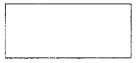(a) square
(b) rectangle
(c) triangle
(d) trapezium.

MCQ On Mensuration For Class 8 Question 2.
The diagram has the shape of a(a) rectangle
(b) square
(c) circle
(d) parallelogram.

Mensuration MCQ Class 8 Question 3.
The diagram has the shape of a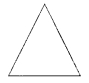(a) circle
(b) rectangle
(c) square
(d) triangle.

MCQ Of Mensuration Class 8 Question 4.
The diagram has the shape of a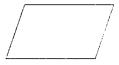(a) rectangle
(b) square
(c) parallelogram
(d) circle.

Class 8 Mensuration MCQ Question 5.
The diagram has the shape of a(a) circle
(b) square
(c) rectangle
(d) parallelogram.

Class 8 Maths Chapter 11 MCQ Question 6.
The diagram has the shape of a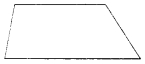(a) circle
(b) parallelogram
(c) rectangle
(d) trapezium.

Class 8 Maths Ch 11 MCQ Question 7.
The area of a rectangle of length a and breadth b is
(a) a + b
(b) ab
(c) a² + b²
(d) 2ab.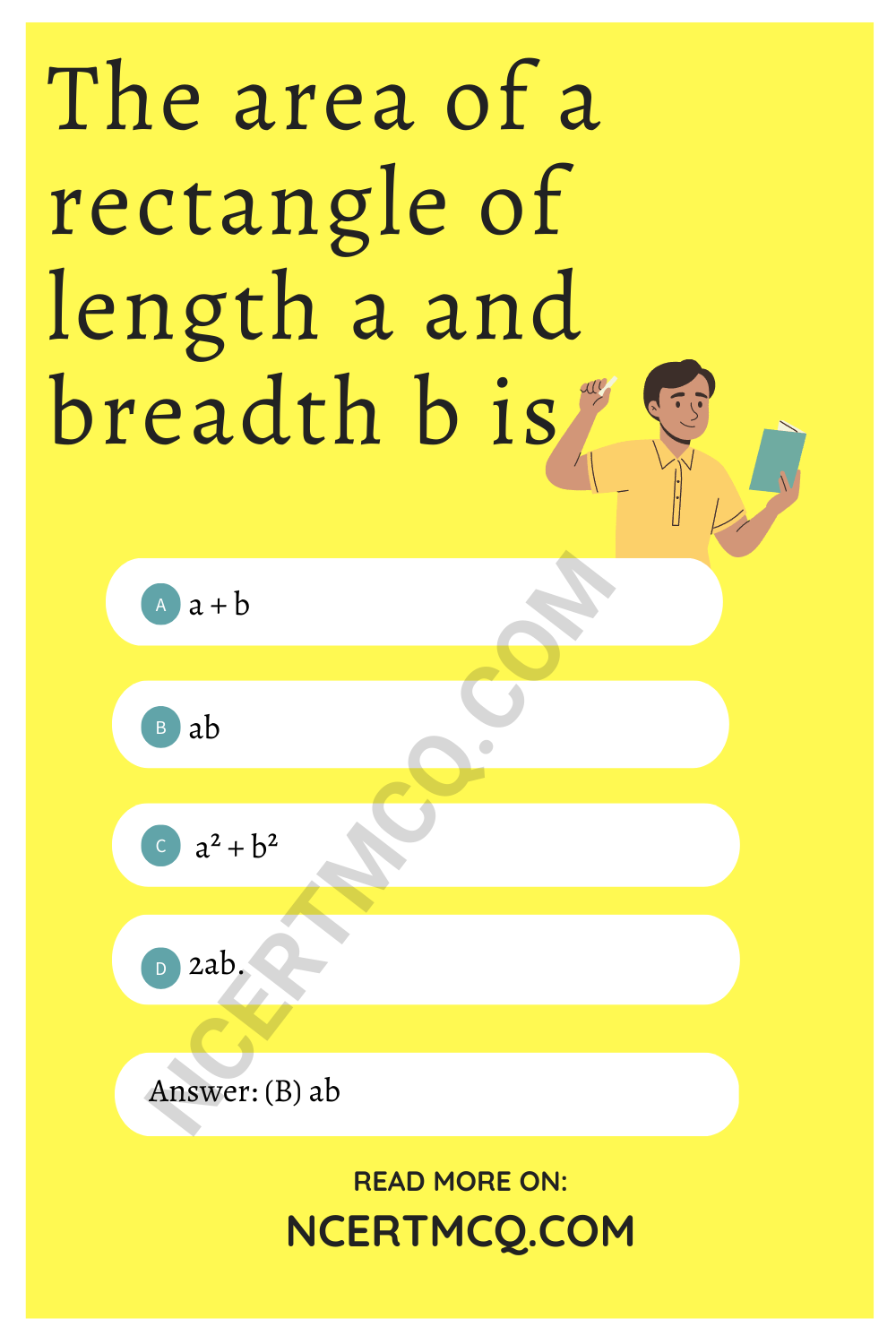Class 8 Maths Mensuration MCQ Question 8.
The area of a square of side a is
(a)a
(b) a²
(c) 2a
(d) 4a.

MCQ Mensuration Class 8 Question 9.
The area of a triangle with base b and altitude h is
(a) $$\frac{1}{2}$$bh
(b) bh
(c) $$\frac{1}{3}$$bh
(d) $$\frac{1}{4}$$bh.

Answer: (a) $$\frac{1}{2}$$bh

Mensuration Class 8 Worksheets MCQ Question 10.
The area of a parallelogram of base b and altitude h is
(a) $$\frac{1}{2}$$bh
(b) bh
(c) $$\frac{1}{3}$$bh
(d) $$\frac{1}{4}$$bh.

MCQs On Mensuration Class 8 Question 11.
The area of a circle of radius r is
(a) $$\frac{1}{2}$$πr²
(b) r²
(c) πr².
(d) $$\frac{1}{4}$$πr²

Ncert Class 8 Maths Chapter 11 MCQ Question 12.
1 cm³ =
(a) 1000 mm³
(b) 100 mm³
(c) 10 mm³
(d) $$\frac{1}{1000}$$mm³

Mensuration Class 8 Extra Questions Question 13.
1 m³ =
(a ) 1000000 cm³
(b) 100 cm³
(c) 10 cm³
(d) $$\frac{1}{1000}$$ cm³

MCQ Questions For Class 8 Maths Mensuration Question 14.
1 mm³ =
(a) 0.001 cm³
(b) 0.01 cm³
(c) 0.1 cm³
(d) 1000 cm³

MCQs Of Mensuration Class 8 Question 15.
1 cm³ =
(a) 0.000001 m³
(b) 0.01 m³
(c) 0.1 m³
(d) 1000 m³

Mensuration Class 8 MCQ Questions Question 16.
The surface area of a cuboid of length l, breadth b and height h is
(a ) lbh
(b) lb + bh + hl
(c) 2 (lb + bh + hl)
(d) 2(l + b)h.

Answer: (c) 2 (lb + bh + hl)

Class 8 Math Chapter 11 MCQ Question 17.
The surface area of a cube of edge a is
(a) 4a²
(b) 6a²
(c) 3a²
(d) a².

Question 18.
The total surface area of a cylinder of base radius r and height h is
(a) 2πr (r + h)
(b) πr (r + h)
(c) 2πrh
(d) 2πr².

Answer: (a) 2πr (r + h)

Question 19.
The volume of a cuboid of length l, breadth b and height h is
(a) lbh
(b) lb + bh + hl
(c) 2 (lb + bh + hl)
(d) 2 (l + b) h.

Question 20.
The volume of a cube of edge a is
(a) a²
(b) a³
(c) a4
(d) 6a²

Question 21.
The volume of a cylinder of base radius r and height h is
(a) 2πrh
(b) πr²h
(c) 2πr (r + h)
(d) $$\frac{1}{3}$$ πr²h.

Question 22.
1 L =
ia) 10 cm³
(b) 100 cm³
(c) 1000 cm³
(d) 10000 cm³.

Question 23.
1 m³ =
(a) 1 L
(b) 10 L
(c) 100 L
(d) 1000 L.

Question 24.
The perimeter of the figure is(a) 7 cm
(b) 14 cm
(c) 12 cm
(d) 24 cm.

Hint:
Perimeter = 2 (4 + 3) = 14 cm.

Question 25.
The area of the figure is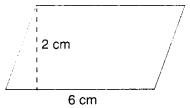(a) 8 cm²
(b) 6 cm²
(c) 12 cm²
(d) 16 cm²

Hint:
Area = 6² = 12 cm².

Question 26.
The perimeter of the figure is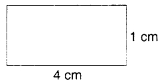(a) 5 cm
(b) 10 cm
(c) 4 cm
(d) 8 cm.

Hint:
Perimeter = 2 (4 + 1) = 10 cm.

Question 27.
The area of the figure is(a) 6 cm²
(b) 12 cm²
(c) 5 cm²
(d) 10 cm²

Hint:
Area = 3 × 2 = 6 cm².

Question 28.
The perimeter of the figure is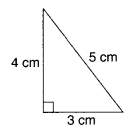(a) 12 cm
(b) 24 cm
(c) 6 cm
(d) 60 cm.

Hint:
Perimeter = 4 + 3 + 5 = 12 cm.

Question 29.
The area of the figure is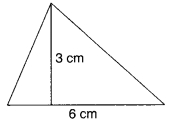(a) 9 cm²
(b) 18 cm²
(c) 12 cm²
(d) 15 cm²

Hint:
Area = $$\frac{6×3}{2}$$ 9 cm².

Question 30.
The perimeter of the figure is(a) 4 cm
(b) 6 cm
(c) 8 cm
(d) 12 cm.

Hint:
Perimeter = 4 × 2 = 8 cm.

Question 31.
The area of the figure is(a) 16 cm²
(b) 8 cm²
(c) 4 cm²
(d) 12 cm²

Hint:
Area = 4 × 4 = 16 cm².

Question 32.
The area of the figure is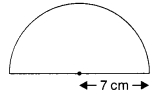(a) 77 cm²
(b) 154 cm²
(c) 38.5 cm²
(d) none of these

Hint:
Area = $$\frac{1}{2}$$ × $$\frac{22}{7}$$ × 7 × 7 = 77 cm².

Question 33.
The area of the trapezium is(a) 9 cm²
(b) 6 cm²
(c) 7 cm²
(d) 24 cm²

Hint:
Area = $$\frac{(4+2)3}{2}$$ = 9 cm².

Question 34.
The area of the trapezium is(a) 6 cm²
(b) 4 cm²
(c) 3 cm²
(d) 9 cm²

Hint:
Area = $$\frac{(3+2)3}{2}$$ = 6 cm².

Question 35.
The perimeter of the trapezium is(a) 12 cm
(b) 24 cm
(c) 6 cm
(d) 18 cm.

Hint:
Perimeter = 3 + 3 + 2 + 4 = 12 cm.

Question 36.
The area of the quadrilateral is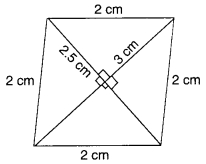(a) 3.75 cm²
(b) 7.5 cm²
(c) 3 cm²
(d) 10 cm²

Hint:
Area = $$\frac{1}{2}$$ × 3 × 2.5 = 1.5 × 2.5
= 3.75 cm².

Question 37.
The area of the quadrilateral is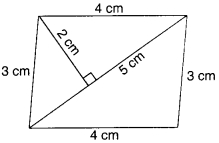(a) 10 cm²
(b) 5 cm²
(c) 20 cm²
(d) 15 cm²

Hint:
Area = 2($$\frac{5×2}{2}$$) = 10 cm².

Question 38.
The area of the quadrilateral is(a) 6 cm²
(b) 12 cm²
(c) 3 cm²
(d) 8 cm²

Hint:
Area = $$\frac{4×(1+2)}{2}$$) = 6 cm²

Question 39.
The area of a rhombus is 60 cm². One diagonal is 10 cm. The other diagonal is
(a) 6 cm
(b) 12 cm
(c) 3 cm
(d) 24 cm.

Hint:
$$\frac{1}{2}$$ × 10 × d2 = 60 ⇒ d2 = 12 cm.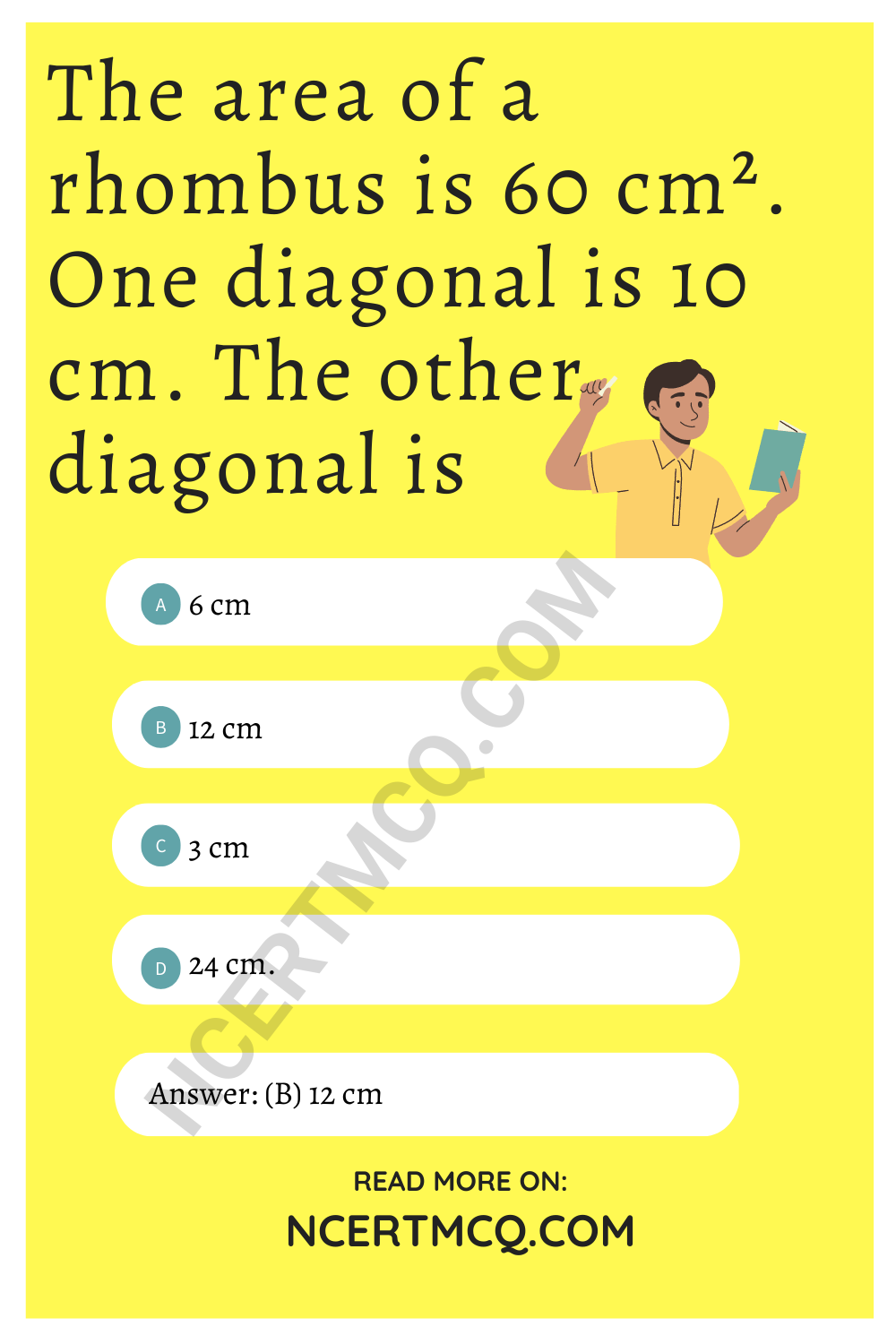Question 40.
The area of a trapezium is 40 cm². Its parallel sides are 12 cm and 8 cm. The distance between the parallel sides is
(a) 1 cm
(b) 2 cm
(c) 3 cm
(d) 4 cm.

Hint:
$$\frac{(12+8)d}{2}$$ = 40 ⇒ d = 4 cm.

Question 41.
8 persons can stay in a cubical room. Each person requires 27 m³ of air. The side of the cube is
(a) 6 m
(b) 4 m
(c) 3 m
(d) 2 m.

Hint:
Volume = 8 × 27 = 216 m³
∴ side = $$\sqrt{216}$$ = 6 m.

Question 42.
If the height of a cuboid becomes zero, it will take the shape of a
(a) cube
(b) parallelogram
(c) circle
(d) rectangle.

Question 43.
The volume of a room is 80 m³. The area of the floor is 20 m². The height of the room is
(a) 1 m
(b) 2 m
(c) 3 m
(d) 4 m.

Hint:
Height = $$\frac{80}{20}$$ = 4 m.

Question 44.
The floor of a room is a square of side 6 m. Its height is 4 m. The volume of the room is
(a) 140 m³
(b) 142 m³
(c) 144 m³
(d) 145 m³

Hint:
Volume = 6 × 6 × 4 = 144 m³.

Question 45.
The base radius and height of a right circular cylinder are 14 cm and 5 cm respectively. Its curved surface is
(a) 220 cm²
(b) 440 cm²
(c) 1232 cm²
(d) 2π × 14 × (14 + 5) cm²

Hint:
Curved surface = 2 × $$\frac{22}{7}$$ × 14 × 5
= 440 cm².

Question 46.
The heights of two right circular cylinders are the same. Their volumes are respectively 16π m³ and 81π m³. The ratio of their base radii is
(a) 16 : 81
(b) 4 : 9
(c) 2 : 3
(d) 9 : 4.

Hint:
$$\frac{πr_1^2h}{πr_2^2h}$$ = $$\frac{16π}{81π}$$ ⇒ $$\frac{r_1}{r_2}$$ = $$\frac{4}{9}$$

Question 47.
The ratio of the radii of two right circular cylinders is 1 : 2 and the ratio of their heights is 4 : 1. The ratio of their volumes is
(a) 1 : 1
(b) 1 : 2
(c) 2 : 1
(d) 4 : 1.

Hint:
$$\frac{r_1}{r_2}$$ = $$\frac{π(1)^24}{π(2)^21}$$ = 1 : 1

Question 48.
A glass in the form of a right circular cylinder is half full of water. Its base radius is 3 cm and height is 8 cm. The volume of water is
(a) 18π cm³
(b) 36π cm³
(c) 9π cm³
(d) 36 cm³

Hint:
Volume = $$\frac{1}{2}$$π × 3 × 3 × 8 = 36π cm³.

Question 49.
The base area of a right circular cylinder is 16K cm³. Its height is 5 cm. Its curved surface area is
(a) 40π cm²
(b) 30π cm²
(c) 20π cm²
(d) 10π cm²

Hint:
πr² = 16π ⇒ r = 4 cm
∴ Curved surface area
= 2 × π × 4 × 5 = 40π cm²

Question 50.
The base radius and height of a right circular cylinder are 5 cm and 10 cm. Its total surface area is
(a) 150π cm²
(b) 300π cm²
(c) 150 cm²
(d) 300 cm²

Hint:
Total surface area = 2πr (h + r)
= 2π 5 (10 + 5) = 150π cm².

Question 51.
If the length and breadth of a rectangle are 10 cm and 5 cm, respectively, then its area is:
(a) 100 sq.cm
(b) 150 sq.cm
(c) 115 sq.cm
(d) 200 sq.cm

Hint:
Length = 10 cm
Area of rectangle = Lenght x breadth
= 10 x 5
= 150 cm2

Question 52.
The area of a rhombus whose diagonals are of lengths 10 cm and 8.2 cm is:
(a) 41 cm2
(b) 82 cm2
(c) 410 cm2
(d) 820 cm2

Hint:
Area of rhombus = ½ d1 d2
A = ½ x 10 x 8.2
A = 41 cm2

Question 53.
The area of a trapezium is 480 cm2, the distance between two parallel sides is 15 cm and one of the parallel side is 20 cm. The other parallel side is:
(a) 20 cm
(b) 34 cm
(c) 44 cm
(d) 50 cm

Hint:
Area of trapezium = ½ h (a + b)
a=20 cm, h = 15 cm, Area = 480 sq.cm
480 = ½ (15) (20 + b)
20 + b = (480 x 2)/15
20 + b = 64
b = 44cm

Question 54.
The area of a rhombus is 240 cm2 and one of the diagonals is 16 cm. Find the other diagonal.
(a) 16 cm
(b) 20 cm
(c) 30 cm
(d) 36 cm

Hint:
Area = 240 cm2
d1 = 16 cm
Area of rhombus = ½ d1 x d2
240 = ½ x 16 x d2
d2 = 480/16 = 30 cm

Question 55.
A cuboid has ______ pairs of identical faces.
(a) 2
(b) 3
(c) 4
(d) 5

Hint:
All six faces are rectangular, and opposites faces are identical. So there are three pairs of identical faces.

Question 56.
All six faces of a cube are:
(a) Identical
(b) Different
(c) Circular
(d) Rectangular

Hint:
All six faces are squares and identical

Question 57.
A cylindrical box has ____ curved surface and ____ circular faces, which are identical.
(a) One, One
(b) One, two
(c) two, one
(d) two, two

Hint:
A cylindrical box having circular bases have identical top. One curved surface and two circular faces which are identical.

Question 58.
If a cuboidal box has height, length and width as 20 cm, 15 cm and 10 cm respectively. Then its total surface area is:
(a) 1100 cm2
(b) 1200 cm2
(c) 1300 cm2
(d) 1400 cm2

Hint:
Total surface area = 2 (20 × 15 + 20 × 10 + 10 × 15)
TSA = 2 ( 300 + 200 + 150) = 1300 cm2

Question 59.
The height of a cylinder whose radius is 7 cm and the total surface area is 968 cm2 is:
(a) 15 cm
(b) 17 cm
(c) 19 cm
(d) 21 cm

Hint:
Total surface area = 2πr (h + r)
968 = 2 x 22/7 x 7 (7+h)
h = 15 cm

Question 60.
The height of a cuboid whose volume is 275 cm3 and base area is 25 cm2 is:
(a) 10 cm
(b) 11 cm
(c) 12 cm
(d) 13 cm